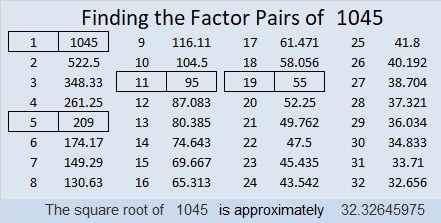# 1045 and Level 2

This puzzle consists of six sets of three numbers. Find the common factor of each set of clues so that ALL of the factors involved are a number from 1 to 12, and you’ll solve this puzzle. Have fun!Here are a few facts about the number 1045:

Obviously, 1045 can be evenly divided by 5, but since 1-0+4-5 = 0, we know that 1045 is also divisible by 11.

• 1045 is a composite number.
• Prime factorization: 1045 = 5 × 11 × 19
• The exponents in the prime factorization are 1, 1, and 1. Adding one to each and multiplying we get (1 + 1)(1 + 1)(1 + 1) = 2 × 2 × 2 = 8. Therefore 1045 has exactly 8 factors.
• Factors of 1045: 1, 5, 11, 19, 55, 95, 209, 1045
• Factor pairs: 1045 = 1 × 1045, 5 × 209, 11 × 95, or 19 × 55
• 1045 has no square factors that allow its square root to be simplified. √1045 ≈ 32.326461045 is the hypotenuse of a Pythagorean triple:
627-836-1045 which is (3-4-5) times 209.

1045 is also palindrome 171 in BASE 29 because 1(29²) + 7(29) + 1(1) = 1045